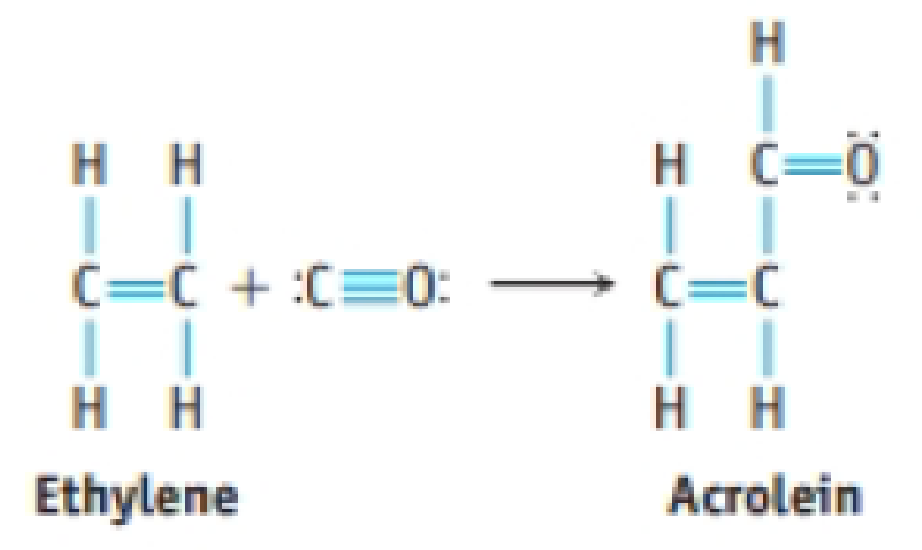Chapter 8, Problem 85GQ

Chapter
Section
Textbook Problem

Acrolein is used to make plastics. Suppose this compound can be prepared by inserting a carbon monoxide molecule into the C—H bond of ethylene.(a) Which is the stronger carbon-carbon bond in acrolein? (b) Which is the longer carbon-carbon bond in acrolein? (c) Is ethylene or acrolein polar? (d) Use bond dissociation enthalpies to predict whether the reaction of CO with C2H4 to give acrolein is endothermic or exothermic.

(a)

Interpretation Introduction

Interpretation: The stronger carbon–carbon bond in acrolein should be identified.

Concept Introduction:

The bond order gives an idea about the stability of a molecule. It can be calculated using the molecular orbital theory. The stability of a molecule increase as the bond order increases.

Bondorder=No. of shared pairs in all X-Y bondsNo. of X-Y links in the molecule or ion

Explanation

The given molecule acrolein contains two type of carbon–carbon bonds that is C-C and C=C bond. Among the bonds C=C is stronger than the C-C bond since

(b)

Interpretation Introduction

Interpretation: The longer carbon–carbon bond in acrolein should be identified.

Concept Introduction:

Bond length:

Bond distance or bond length is the average distance between nuclei of two bonded atoms in a molecule. The bond length and bond order are inversely proportional hence bond length increases the bond order decreases.

Bond Order:

The bond order gives an idea about the stability of a molecule. It can be calculated using the molecular orbital theory. The stability of a molecule increase as the bond order increases.

Bondorder=No. of shared pairs in all X-Y bondsNo. of X-Y links in the molecule or ion

(c)

Interpretation Introduction

Interpretation: The longer carbon–carbon bond in acrolein should be identified.

Concept Introduction:

Electronegativity: It is defined as the capacity of the atom to abstract the pair of electrons towards itself results to have high negative charge.

Polar molecule: The molecule with atoms bonded with different electronegativity. Dipole moment is used to measure the polarity of the molecule.

Polarity of a molecule is measured in term of dipole moment.

Dipole moment for a polar molecule is non-zero and for a non-polar molecule dipole moment is zero.

(d)

Interpretation Introduction

Interpretation: The reaction of CO with C2H4 that result in acrolein should be identified that whether the reaction is endothermic or exothermic using bond dissociation enthalpies.

Concept Introduction:

Bond dissociation enthalpy:

Bond energy or more correctly the bond dissociation enthalpy is the enthalpy change when breaking a bond in a molecule with the reactant and products in the gas phase.

The process of breaking bonds in a molecule is always endothermic, so ΔrH for bond breaking is always positive.

ΔrH0 = ΔH(bonds broken)-ΔH(bonds formed)

Still sussing out bartleby?

Check out a sample textbook solution.

See a sample solution

The Solution to Your Study Problems

Bartleby provides explanations to thousands of textbook problems written by our experts, many with advanced degrees!

Get Started